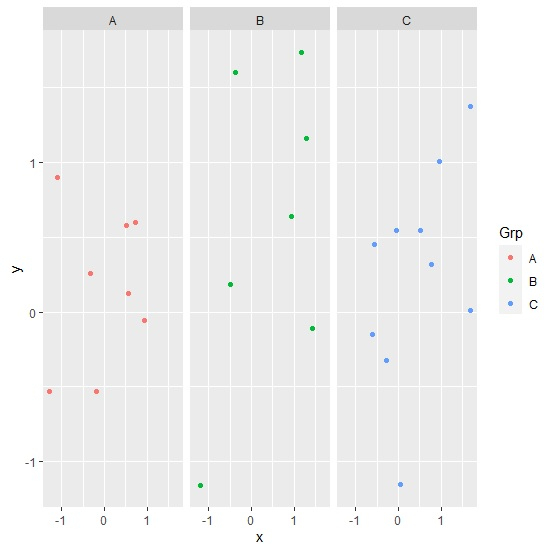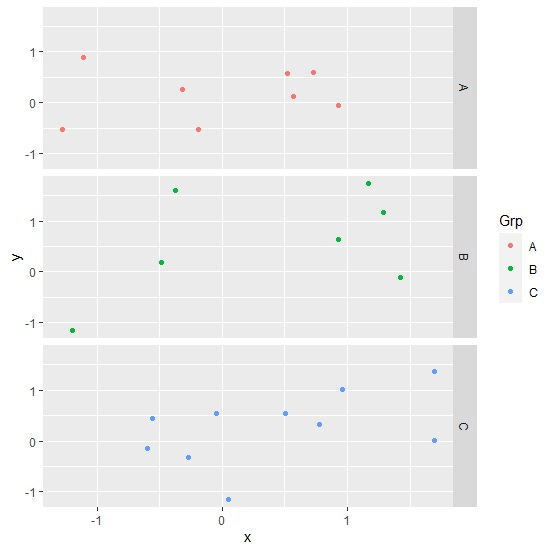# How to create facetted plot with facets in horizontal direction using ggplot2 in R?

To create facetted plot with facets in horizontal direction using ggplot2 in R, we can follow the below steps −

• First of all, create a data frame.
• Then, create the facetted plot using facet_grid function of ggplot2 package.
• Use facet_grid function with reversed value of categorical column to create the facetted plot with facets in horizontal direction.

## Create the data frame

Let's create a data frame as shown below −

Live Demo

x<-rnorm(25)
y<-rnorm(25)
Grp<-sample(LETTERS[1:3],25,replace=TRUE)
df<-data.frame(x,y,Grp)
df

On executing, the above script generates the below output(this output will vary on your system due to randomization) −

         x             y   Grp
1   0.04783437  -1.15182325 C
2   0.56696771  0.12167256 A
3  -1.19615960 -1.16038178 B
4   0.95960082  1.00415819 C
5   0.50725210  0.54469494 C
6  -0.37411224  1.60231699 B
7   1.42044733 -0.11057038 B
8   1.69437460  0.01264523 C
9   0.51677914  0.57436245 A
10 -0.04810441  0.54283138 C
11 -1.28024577 -0.53045053 A
12 -0.48968148  0.18115294 B
13  1.28769546  1.16062792 B
14  0.93050145  0.63649217 B
15  0.72614070  0.59524893 A
16 -0.19306114 -0.52972220 A
17 -0.56122938  0.45013366 C
18  0.77504861  0.31892391 C
19 -0.26756380 -0.32371074 C
20 -1.11077206  0.89716628 A
21 -0.60040090 -0.15187155 C
22 -0.31920172  0.25808226 A
23  1.16802977 1.73149549 B
24  1.69736349 1.36917698 C
25  0.93134640 -0.05803692 A

## Create the facetted plot

Use facet_grid function to create point chart between x and y based on Grp column −

x<-rnorm(25)
y<-rnorm(25)
Grp<-sample(LETTERS[1:3],25,replace=TRUE)
df<-data.frame(x,y,Grp)
library(ggplot2)
ggplot(df,aes(x,y,col=Grp))+geom_point()+facet_grid(~Grp)

### Output## Create the facetted plot with facets in horizontal direction

Use facet_grid function to create point chart between x and y based on Grp column by providing Grp column name before tilde as shown below −

x<-rnorm(25)
y<-rnorm(25)
Grp<-sample(LETTERS[1:3],25,replace=TRUE)
df<-data.frame(x,y,Grp)
library(ggplot2)
ggplot(df,aes(x,y,col=Grp))+geom_point()+facet_grid(Grp~.)

### Output